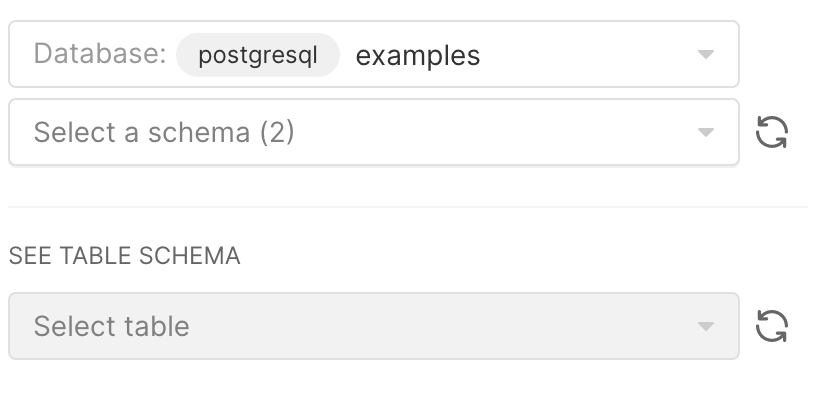Table Joins
• 06 Jul 2022
• Dark
Light

# Table Joins

• Dark
Light

Article Summary

## Overview

When writing query joins in SQL lab, there are several restrictions:

1. Joined tables must be from the same database.
2. Joined tables can be from different schemas within a database.
3. Joined tables can be from the same schema within a database.

## Examples

### Example for Restriction 1

There exists a table called public.example_one in a Postgresql database called post and another table called public.example_two in an Athena database called ath.

A SQL query such as:

``````SELECT * FROM post.public.example_one
JOIN ath.public.example_two ON example_one.id = example_two.id
``````

...will be invalid. Even if the two databases were both Postgresql databases, you will not be able to join. Tables must originate from the same database.

### Example for Restriction 2

There exists a table called schema_one.example_one and another table called schema_two.example_two in the same database.

A SQL query such as:

``````SELECT * FROM schema_one.example_one
JOIN schema_two.example_two ON example_one.id = example_two.id
``````

...will be valid.

### Example for Restriction 3

There exists a table called public.example_one and another table called public.example_two in the same database.

A SQL query such as:

``````SELECT * FROM public.example_one
JOIN public.example_two ON example_one.id = example_two.id
``````

...will be valid.

#### Specify the database you are using in SQL Lab

From the SQL Editor, in the Database field, ensure that you specify the database that contains your tables/schemas.